• 读取视频序列
• 处理视频帧
• 编写视频序列
• 跟踪视频中的特征点
• 提取视频中的前景对象

# 读取视频序列

## 操作步骤

int main()
{
// Open the video file
cv::VideoCapture capture("../bike.avi");
// check if video successfully opened
if (!capture.isOpened())
return 1;

// Get the frame rate
double rate= capture.get(CV_CAP_PROP_FPS);

bool stop(false);
cv::Mat frame; // current video frame
cv::namedWindow("Extracted Frame");

// Delay between each frame in ms
// corresponds to video frame rate
int delay= 1000/rate;

// for all frames in video
while (!stop) {

// read next frame if any
break;

cv::imshow("Extracted Frame",frame);

// introduce a delay
// or press key to stop
if (cv::waitKey(delay)>=0)
stop= true;
}

// Close the video file.
// Not required since called by destructor
capture.release();
}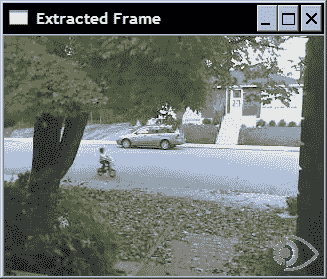## 工作原理

long t= static_cast<long>(
capture.get(CV_CAP_PROP_FRAME_COUNT));


// goto frame 100
double position= 100.0;
capture.set(CV_CAP_PROP_POS_FRAMES, position);


capture >> frame;


capture.grab();
capture.retrieve(frame);


## 另见

ffmpeg.org网站提供了一个完整的开源和跨平台解决方案，用于音频/视频读取，记录，转换和流传输。 用于操纵视频文件的 OpenCV 类是在此库之上构建的。

Xvid.org网站提供了一个基于 MPEG-4 标准的开源视频编解码器库，用于视频压缩。 Xvid 还有一个竞争对手 DivX ，它提供专有但免费的编解码器和软件工具。

# 处理视频帧

## 操作步骤

void processFrame(cv::Mat& img, cv::Mat& out);


void canny(cv::Mat& img, cv::Mat& out) {

// Convert to gray
if (img.channels()==3)
cv::cvtColor(img,out,CV_BGR2GRAY);
// Compute Canny edges
cv::Canny(out,out,100,200);
// Invert the image
cv::threshold(out,out,128,255,cv::THRESH_BINARY_INV);
}


   // Create instance
VideoProcessor processor;
// Open video file
processor.setInput("../bike.avi");
// Declare a window to display the video
processor.displayInput("Current Frame");
processor.displayOutput("Output Frame");
// Play the video at the original frame rate
processor.setDelay(1000./processor.getFrameRate());
// Set the frame processor callback function
processor.setFrameProcessor(canny);
// Start the process
processor.run();


class VideoProcessor {

private:

// the OpenCV video capture object
cv::VideoCapture capture;
// the callback function to be called
// for the processing of each frame
void (*process)(cv::Mat&, cv::Mat&);
// a bool to determine if the
// process callback will be called
bool callIt;
// Input display window name
std::string windowNameInput;
// Output display window name
std::string windowNameOutput;
// delay between each frame processing
int delay;
// number of processed frames
long fnumber;
// stop at this frame number
long frameToStop;
// to stop the processing
bool stop;

public:

VideoProcessor() : callIt(true), delay(0),
fnumber(0), stop(false), frameToStop(-1) {}


     // set the callback function that
// will be called for each frame
void setFrameProcessor(
void (*frameProcessingCallback)
(cv::Mat&, cv::Mat&)) {

process= frameProcessingCallback;
}


     // set the name of the video file
bool setInput(std::string filename) {

fnumber= 0;
// In case a resource was already
// associated with the VideoCapture instance
capture.release();
images.clear();

// Open the video file
return capture.open(filename);
}


     // to display the processed frames
void displayInput(std::string wn) {

windowNameInput= wn;
cv::namedWindow(windowNameInput);
}

// to display the processed frames
void displayOutput(std::string wn) {

windowNameOutput= wn;
cv::namedWindow(windowNameOutput);
}

// do not display the processed frames
void dontDisplay() {

cv::destroyWindow(windowNameInput);
cv::destroyWindow(windowNameOutput);
windowNameInput.clear();
windowNameOutput.clear();
}


     // to grab (and process) the frames of the sequence
void run() {

// current frame
cv::Mat frame;
// output frame
cv::Mat output;

// if no capture device has been set
if (!isOpened())
return;

stop= false;

while (!isStopped()) {

// read next frame if any
break;

// display input frame
if (windowNameInput.length()!=0)
cv::imshow(windowNameInput,frame);

// calling the process function
if (callIt) {

// process the frame
process(frame, output);
// increment frame number
fnumber++;

} else {

output= frame;
}

// display output frame
if (windowNameOutput.length()!=0)
cv::imshow(windowNameOutput,output);

// introduce a delay
if (delay>=0 && cv::waitKey(delay)>=0)
stopIt();

// check if we should stop
if (frameToStop>=0 &&
stopIt();
}
}

// Stop the processing
void stopIt() {

stop= true;
}

// Is the process stopped?
bool isStopped() {

return stop;
}

// Is a capture device opened?
bool isOpened() {

capture.isOpened();
}

// set a delay between each frame
// 0 means wait at each frame
// negative means no delay
void setDelay(int d) {

delay= d;
}


     // to get the next frame
// could be: video file or camera

}


     // process callback to be called
void callProcess() {

callIt= true;
}

// do not call process callback
void dontCallProcess() {

callIt= false;
}


     void stopAtFrameNo(long frame) {

frameToStop= frame;
}

// return the frame number of the next frame

// get info of from the capture device
long fnumber= static_cast<long>(
capture.get(CV_CAP_PROP_POS_FRAMES));
return fnumber;
}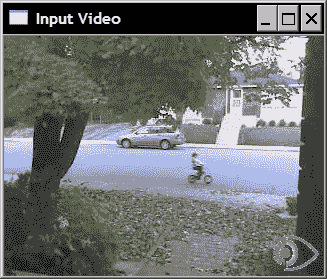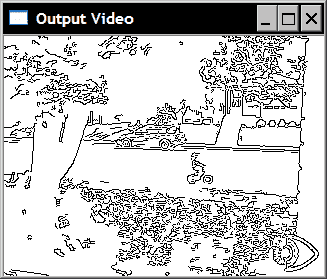## 更多

### 处理图像序列

     // vector of image filename to be used as input
std::vector<std::string> images;
// image vector iterator
std::vector<std::string>::const_iterator itImg;


     // set the vector of input images
bool setInput(const std::vector<std::string>& imgs) {

fnumber= 0;
// In case a resource was already
// associated with the VideoCapture instance
capture.release();

// the input will be this vector of images
images= imgs;
itImg= images.begin();

return true;
}


isOpened方法变为：

     // Is a capture device opened?
bool isOpened() {

return capture.isOpened() || !images.empty();
}


     // to get the next frame
// could be: video file; camera; vector of images

if (images.size()==0)

else {

if (itImg != images.end()) {

itImg++;
return frame.data != 0;

} else {

return false;
}
}
}


### 使用帧处理器类

// The frame processor interface
class FrameProcessor {

public:
// processing method
virtual void process(cv:: Mat &input, cv:: Mat &output)= 0;
};


     // set the instance of the class that
// implements the FrameProcessor interface
void setFrameProcessor(FrameProcessor* frameProcessorPtr)
{

// invalidate callback function
process= 0;
// this is the frame processor instance
// that will be called
frameProcessor= frameProcessorPtr;
callProcess();
}


     // set the callback function that will
// be called for each frame
void setFrameProcessor(
void (*frameProcessingCallback)(cv::Mat&, cv::Mat&)) {

// invalidate frame processor class instance
frameProcessor= 0;
// this is the frame processor function that
// will be called
process= frameProcessingCallback;
callProcess();
}


        while (!isStopped()) {

// read next frame if any
break;

// display input frame
if (windowNameInput.length()!=0)
cv::imshow(windowNameInput,frame);

// ** calling the process function or method **
if (callIt) {

// process the frame
if (process) // if call back function
process(frame, output);
else if (frameProcessor)
// if class interface instance
frameProcessor->process(frame,output);
// increment frame number
fnumber++;

} else {

output= frame;
}

// display output frame
if (windowNameOutput.length()!=0)
cv::imshow(windowNameOutput,output);

// introduce a delay
if (delay>=0 && cv::waitKey(delay)>=0)
stopIt();

// check if we should stop
if (frameToStop>=0 &&
stopIt();
}


# 编写视频序列

## 操作步骤

class VideoProcessor {

private:

...
// the OpenCV video writer object
cv::VideoWriter writer;
// output filename
std::string outputFile;
// current index for output images
int currentIndex;
// number of digits in output image filename
int digits;
// extension of output images
std::string extension;


     // set the output video file
// by default the same parameters than
// input video will be used
bool setOutput(const std::string &filename,
int codec=0, double framerate=0.0,
bool isColor=true) {

outputFile= filename;
extension.clear();

if (framerate==0.0)
framerate= getFrameRate(); // same as input

char c;
// use same codec as input
if (codec==0) {
codec= getCodec(c);
}

// Open output video
return writer.open(outputFile, // filename
codec,          // codec to be used
framerate,      // frame rate of the video
getFrameSize(), // frame size
isColor);       // color video?
}


     // to write the output frame
// could be: video file or images
void writeNextFrame(cv::Mat& frame) {

if (extension.length()) { // then we write images

std::stringstream ss;
// compose the output filename
ss << outputFile << std::setfill('0')
<< std::setw(digits)
<< currentIndex++ << extension;
cv::imwrite(ss.str(),frame);

} else { // then write to video file

writer.write(frame);
}
}


     // set the output as a series of image files
// extension must be ".jpg", ".bmp" ...
bool setOutput(const std::string &filename, // prefix
const std::string &ext, // image file extension
int numberOfDigits=3,   // number of digits
int startIndex=0) {     // start index

// number of digits must be positive
if (numberOfDigits<0)
return false;

// filenames and their common extension
outputFile= filename;
extension= ext;

// number of digits in the file numbering scheme
digits= numberOfDigits;
// start numbering at this index
currentIndex= startIndex;

return true;
}


        while (!isStopped()) {

// read next frame if any
break;

// display input frame
if (windowNameInput.length()!=0)
cv::imshow(windowNameInput,frame);

// calling the process function or method
if (callIt) {

// process the frame
if (process)
process(frame, output);
else if (frameProcessor)
frameProcessor->process(frame,output);
// increment frame number
fnumber++;

} else {

output= frame;
}

// ** write output sequence **
if (outputFile.length()!=0)
writeNextFrame(output);

// display output frame
if (windowNameOutput.length()!=0)
cv::imshow(windowNameOutput,output);

// introduce a delay
if (delay>=0 && cv::waitKey(delay)>=0)
stopIt();

// check if we should stop
if (frameToStop>=0 &&
stopIt();
}
}


   // Create instance
VideoProcessor processor;

// Open video file
processor.setInput("../bike.avi");
processor.setFrameProcessor(canny);
processor.setOutput("../bikeOut.avi");
// Start the process
processor.run();


   processor.setOutput("../bikeOut",".jpg");


## 工作原理

     // get the codec of input video
int getCodec(char codec) {

// undefined for vector of images
if (images.size()!=0) return -1;

union { // data structure for the 4-char code
int value;
char code; } returned;

// get the code
returned.value= static_cast<int>(
capture.get(CV_CAP_PROP_FOURCC));

// get the 4 characters
codec= returned.code;
codec= returned.code;
codec= returned.code;
codec= returned.code;

// return the int value corresponding to the code
return returned.value;
}


get方法始终返回double，然后将其转换为整数。 该整数表示可使用union数据结构从中提取四个字符的代码。 如果我们打开测试视频序列，则从以下语句开始：

   char codec;
processor.getCodec(codec);
std::cout << "Codec: " << codec << codec
<< codec << codec << std::endl;


Codec : XVID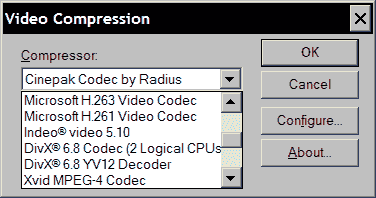# 跟踪视频中的特征点

## 操作步骤

class FeatureTracker : public FrameProcessor {

cv::Mat gray;         // current gray-level image
cv::Mat gray_prev;      // previous gray-level image
// tracked features from 0->1
std::vector<cv::Point2f> points;
// initial position of tracked points
std::vector<cv::Point2f> initial;
std::vector<cv::Point2f> features;  // detected features
int max_count;     // maximum number of features to detect
double qlevel;    // quality level for feature detection
double minDist;   // min distance between two points
std::vector<uchar> status; // status of tracked features
std::vector<float> err;    // error in tracking

public:

FeatureTracker() : max_count(500),
qlevel(0.01), minDist(10.) {}


   void process(cv:: Mat &frame, cv:: Mat &output) {

// convert to gray-level image
cv::cvtColor(frame, gray, CV_BGR2GRAY);
frame.copyTo(output);

// 1\. if new feature points must be added
{
// detect feature points
detectFeaturePoints();
// add the detected features to
// the currently tracked features
points.insert(points.end(),
features.begin(),features.end());
initial.insert(initial.end(),
features.begin(),features.end());
}

// for first image of the sequence
if(gray_prev.empty())
gray.copyTo(gray_prev);

// 2\. track features
cv::calcOpticalFlowPyrLK(
gray_prev, gray, // 2 consecutive images
points, // input point positions in first image
points, // output point positions in the 2nd image
status,    // tracking success
err);      // tracking error

// 2\. loop over the tracked points to reject some
int k=0;
for( int i= 0; i < points.size(); i++ ) {

// do we keep this point?
if (acceptTrackedPoint(i)) {

// keep this point in vector
initial[k]= initial[i];
points[k++] = points[i];
}
}

// eliminate unsuccesful points
points.resize(k);
initial.resize(k);

// 3\. handle the accepted tracked points
handleTrackedPoints(frame, output);

// 4\. current points and image become previous ones
std::swap(points, points);
cv::swap(gray_prev, gray);
}


   // feature point detection
void detectFeaturePoints() {

// detect the features
cv::goodFeaturesToTrack(gray, // the image
features,   // the output detected features
max_count,  // the maximum number of features
qlevel,     // quality level
minDist);   // min distance between two features
}


   // determine if new points should be added

// if too few points
return points.size()<=10;
}


   // determine which tracked point should be accepted
bool acceptTrackedPoint(int i) {

return status[i] &&
// if point has moved
(abs(points[i].x-points[i].x)+
(abs(points[i].y-points[i].y))>2);
}


   // handle the currently tracked points
void handleTrackedPoints(cv:: Mat &frame,
cv:: Mat &output) {

// for all tracked points
for(int i= 0; i < points.size(); i++ ) {

// draw line and circle
cv::line(output,
initial[i],  // initial position
points[i],// new position
cv::Scalar(255,255,255));
cv::circle(output, points[i], 3,
cv::Scalar(255,255,255),-1);
}
}


int main()
{
// Create video procesor instance
VideoProcessor processor;

// Create feature tracker instance
FeatureTracker tracker;

// Open video file
processor.setInput("../bike.avi");

// set frame processor
processor.setFrameProcessor(&tracker);

// Declare a window to display the video
processor.displayOutput("Tracked Features");

// Play the video at the original frame rate
processor.etDelayetDelay(1000./processor.getFrameRate());

// Start the process
processor.run();
}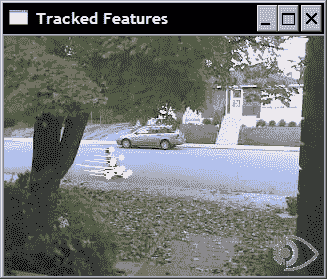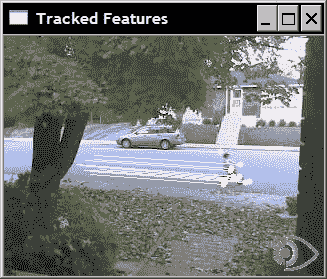## 工作原理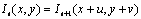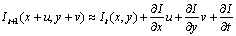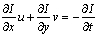## 另见

The classic article by B. Lucas and T. Kanade, An iterative image registration technique with an application to stereo vision in Int. Joint Conference in Artificial Intelligence, pp. 674-679, 1981, that describes the original feature point tracking algorithm.

The article by J. Shi and C. Tomasi, Good Features to Track in IEEE Conference on Computer Vision and Pattern Recognition, pp. 593-600, 1994, that describes an improved version of the original feature point tracking algorithm.


# 提取视频中的前景对象

## 操作步骤

   // compute difference between current image and background
cv::absdiff(backgroundImage,currentImage,foreground);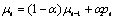class BGFGSegmentor : public FrameProcessor {

cv::Mat gray;         // current gray-level image
cv::Mat background;      // accumulated background
cv::Mat backImage;      // background image
cv::Mat foreground;      // foreground image
// learning rate in background accumulation
double learningRate;
int threshold;         // threshold for foreground extraction

public:

BGFGSegmentor() : threshold(10), learningRate(0.01) {}


   // processing method
void process(cv:: Mat &frame, cv:: Mat &output) {

// convert to gray-level image
cv::cvtColor(frame, gray, CV_BGR2GRAY);

// initialize background to 1st frame
if (background.empty())
gray.convertTo(background, CV_32F);

// convert background to 8U
background.convertTo(backImage,CV_8U);

// compute difference between image and background
cv::absdiff(backImage,gray,foreground);

// apply threshold to foreground image
cv::threshold(foreground,output,
threshold,255,cv::THRESH_BINARY_INV);

// accumulate background
cv::accumulateWeighted(gray, background,
learningRate, output);
}


int main()
{
// Create video procesor instance
VideoProcessor processor;

// Create background/foreground segmentor
BGFGSegmentor segmentor;
segmentor.setThreshold(25);

// Open video file
processor.setInput("../bike.avi");

// set frame processor
processor.setFrameProcessor(&segmentor);

// Declare a window to display the video
processor.displayOutput("Extracted Foreground");

// Play the video at the original frame rate
processor.setDelay(1000./processor.getFrameRate());

// Start the process
processor.run();
}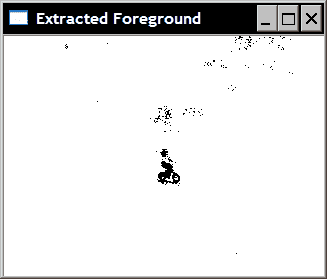## 更多

### 高斯方法的混合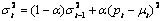int main()
{
// Open the video file
cv::VideoCapture capture("../bike.avi");
// check if video successfully opened
if (!capture.isOpened())
return 0;

// current video frame
cv::Mat frame;
// foreground binary image
cv::Mat foreground;

cv::namedWindow("Extracted Foreground");

// The Mixture of Gaussian object
// used with all default parameters
cv::BackgroundSubtractorMOG mog;

bool stop(false);
// for all frames in video
while (!stop) {

// read next frame if any
break;

// update the background
// and return the foreground
mog(frame,foreground,0.01);

// Complement the image
cv::threshold(foreground,foreground,
128,255,cv::THRESH_BINARY_INV);

// show foreground
cv::imshow("Extracted Foreground",foreground);

// introduce a delay
// or press key to stop
if (cv::waitKey(10)>=0)
stop= true;
}
}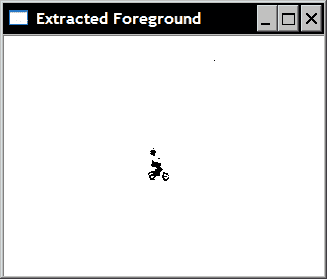## 另见

The article by C. Stauffer and W.E.L. Grimson, Adaptive background mixture models for real-time tracking, in Conf. on Computer Vision and Pattern Recognition, 1999, for a more complete description of the Mixture of Gaussian algorithm.